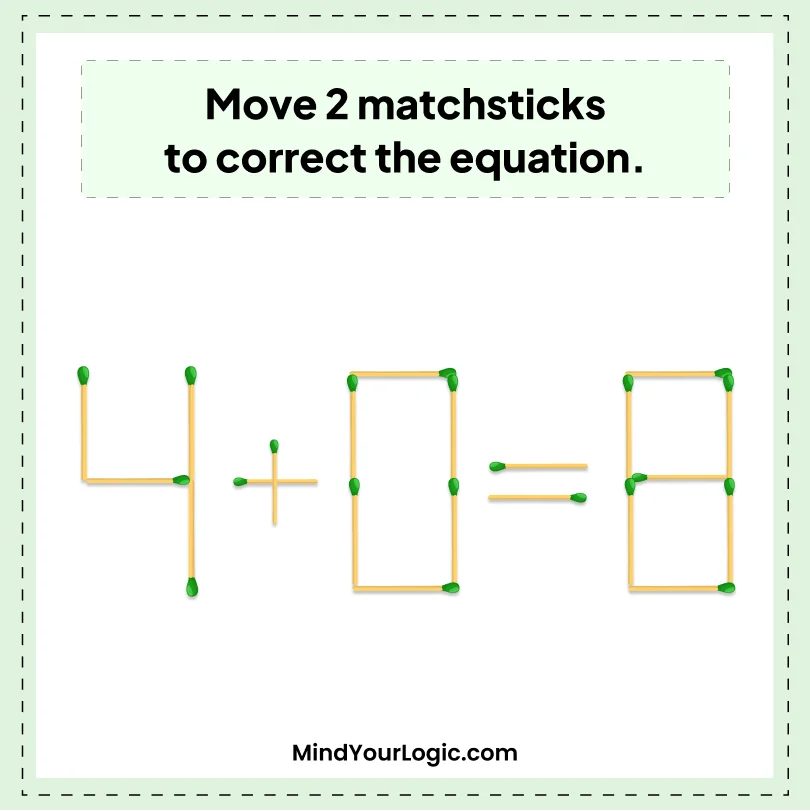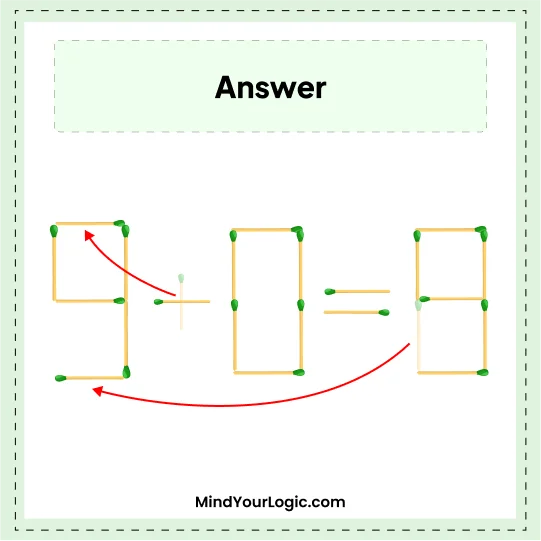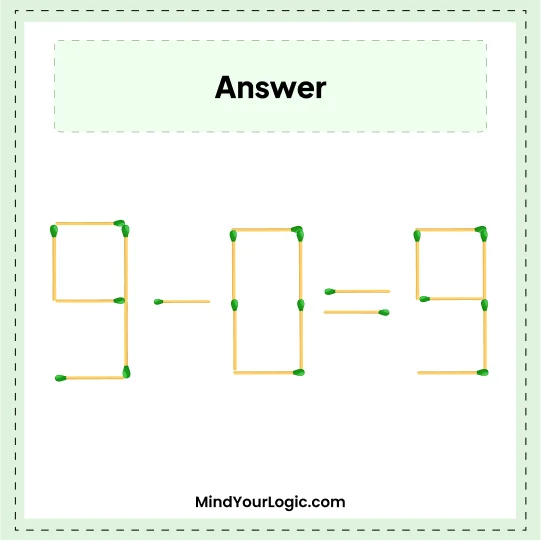# 4+0=8 - Matchstick puzzle

###### 36.Matchstick Puzzles
```Move 2 Matchsticks to get correct equation.
```•

Explanation :

` Move the sticks of 8 and + sign to 4 and create it 9.Therefore, the equation will look like  9 + 0  =  9.`